# 1. (9 points) Balance the following reactions: H3PO4(1) + HCIE) C4H3 + O2 CO2 + H2O...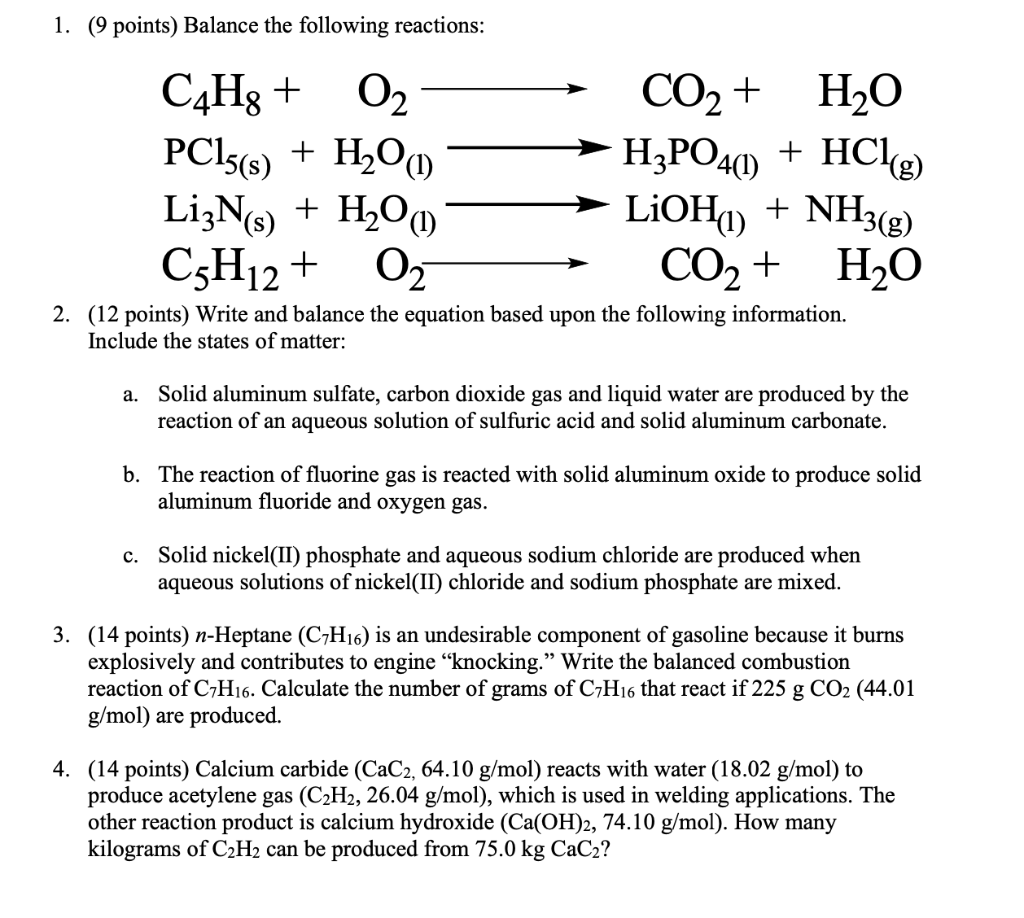1. (9 points) Balance the following reactions: H3PO4(1) + HCIE) C4H3 + O2 CO2 + H2O PC15(s) + H2O LizNs) LiOH) + NH3(g) C3H12 + O2 CO2 + H2O 2. (12 points) Write and balance the equation based upon the following information. + H2O(1) Include the states of matter: a. Solid aluminum sulfate, carbon dioxide gas and liquid water are produced by the reaction of an aqueous solution of sulfuric acid and solid aluminum carbonate. b. The reaction of fluorine gas is reacted with solid aluminum oxide to produce solid aluminum fluoride and oxygen gas. c. Solid nickel(II) phosphate and aqueous sodium chloride are produced when aqueous solutions of nickel(II) chloride and sodium phosphate are mixed. 3. (14 points) n-Heptane (C7H16) is an undesirable component of gasoline because it burns explosively and contributes to engine “knocking.” Write the balanced combustion reaction of C7H16. Calculate the number of grams of C7H16 that react if 225 g CO2 (44.01 g/mol) are produced. 4. (14 points) Calcium carbide (CaC2, 64.10 g/mol) reacts with water (18.02 g/mol) to produce acetylene gas (C2H2, 26.04 g/mol), which is used in welding applications. The other reaction product is calcium hydroxide (Ca(OH)2, 74.10 g/mol). How many kilograms of C2H2 can be produced from 75.0 kg CaCz?

1)

a)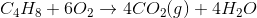b)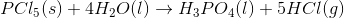c)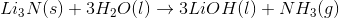d)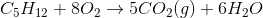2)

a)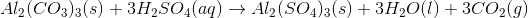b)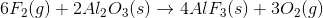c)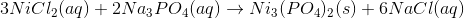3)

Combustion of n- heptane is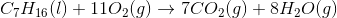Molar mass of n-heptane is 7*12+16*1 = 100 g/mol

100 g of n-heptane on Combustion gives 7*44 g of Carbon dioxide.

How many grams of n-heptane on Combustion gives 225 g of carbon dioxide.

= 225 g * 100 g/7 *44 g

= 73.05 g

4)

Hydrolysis of calcium carbide is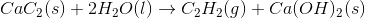Molar mass of Calcium carbide is 1*40+2*12 = 64 g/mol

Molar mass of acetylene = 2*12+2*1 = 26 g/mol

64 g of calcium carbide on Hydrolysis gives 26 g of acetylene.

75000 g of calcium carbide on hydrolysis gives ? g of acetylene.

= 75000 g * 26 g/64 g

= 30468.75 g

= 30.5 Kg

#### Earn Coin

Coins can be redeemed for fabulous gifts.

Similar Homework Help Questions
• ### Consider the following reaction:              C2H2 (g)+ 52 O2 (g) ® 2 CO2 (g) + H2O...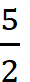Consider the following reaction:              C2H2 (g)+ 52 O2 (g) ® 2 CO2 (g) + H2O (g)                       Given ΔHf° of CO2 (g) = -393.5 KJ/mol, ΔHf° H2O (g) = -241.8 KJ/mol, and ΔHf° for C2H2 (g) = 227.4 KJ/mol, calculate ΔHrxn° for this reaction. How many KJ of heat is released when 0.440 kg of carbon dioxide produced?

• ### 2) Oxyacetylene torches are fueled by the combustion of acetylene, C2H2 2 C2H2 + 5 O2...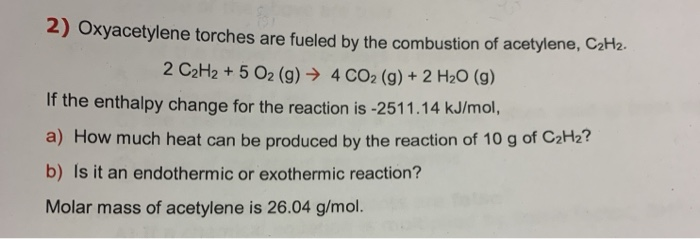2) Oxyacetylene torches are fueled by the combustion of acetylene, C2H2 2 C2H2 + 5 O2 (g) 4 CO2 (g) + 2 H2O (g) If the enthalpy change for the reaction is -251 1.14 kJ/mol, a) How much heat can be produced by the reaction of 10 g of C2H2? b) Is it an endotherm ic or exothermic reaction? Molar mass of acetylene is 26.04 g/mol.

• ### 1).From the following enthalpy changes, S (s) +3/2 O2 (g) 2 SO2 (g) SO3 (g) O2...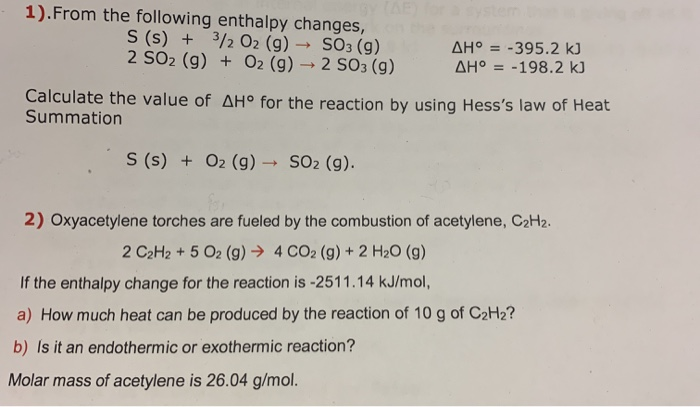1).From the following enthalpy changes, S (s) +3/2 O2 (g) 2 SO2 (g) SO3 (g) O2 (g)2 SO3 (g) AH =-395.2 kJ AHo 198.2 kJ Calculate the value of AHo for the reaction by using Hess's law of Heat Summation S(s) O2 (g) SO2 (g) 2) Oxyacetylene torches are fueled by the combustion of acetylene, C2H2. 4 CO2 (g) +2 H20 (g) 2 C2H2 + 5 O2 (g) If the enthalpy change for the reaction is -2511.14 kJ/mol, a) How...

• ### Acetylene is used in blow torches, and burns according to the following equation: 2 C2H2(g) + 5 O2(g) → 4 CO2(g) + 2 H2O(g) Use the following information to calculate the heat of reaction:?

Acetylene is used in blow torches, and burns according to the following equation: 2 C2H2(g) + 5 O2(g) → 4 CO2(g) + 2 H2O(g) Use the following information to calculate the heat of reaction: Hfo (H2O(g))= -241.82 kJ/mol Hfo (CO2(g))= -393.5 kJ/mol Hfo (C2H2(g))=226.77 kJ/mol

• ### You have the following info: C3H8(t) + O2(g) → CO2(g) + H2O(g) AHrx = -2044 kJ...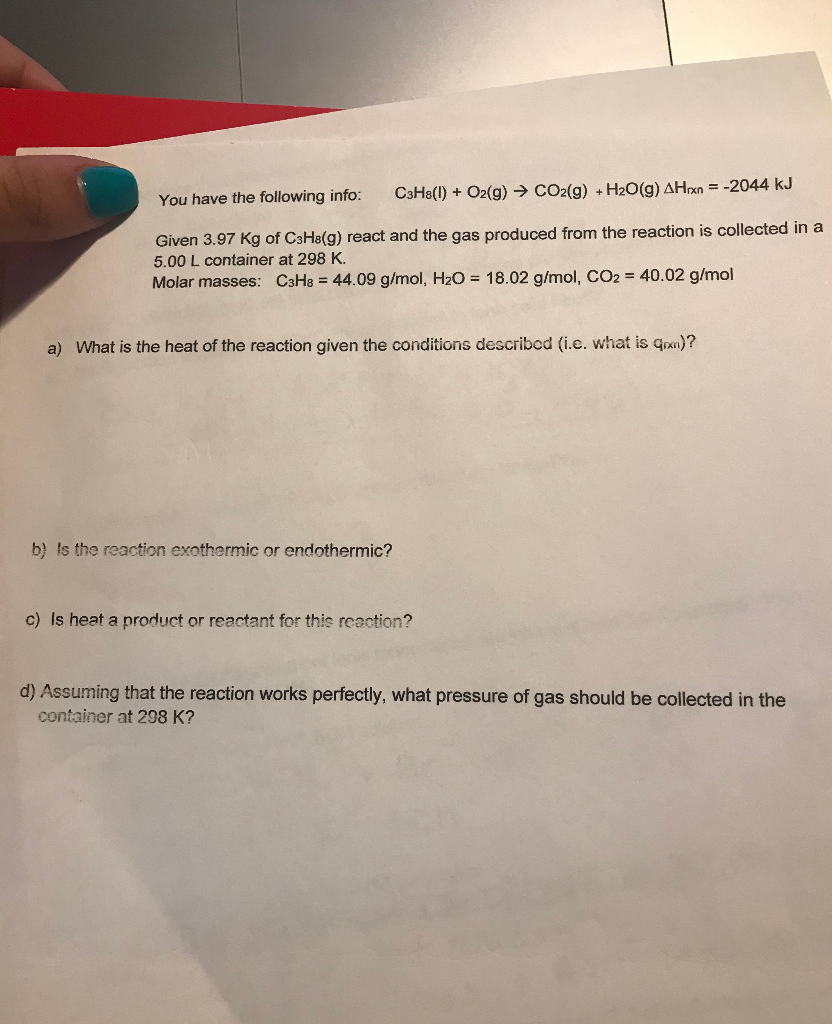You have the following info: C3H8(t) + O2(g) → CO2(g) + H2O(g) AHrx = -2044 kJ Given 3.97 kg of C3H8(9) react and the gas produced from the reaction is collected in a 5.00 L container at 298 K. Molar masses: C3H8 = 44.09 g/mol, H2O = 18.02 g/mol, CO2 = 40.02 g/mol a) What is the heat of the reaction given the conditions described (i.e. what is Gnx)? b) is the reaction exothermic or endothermic? c) Is heat a...

• ### 52. For the reaction of C2H4(g) with O2(g) to form CO2(g) and H2O(g), what number of...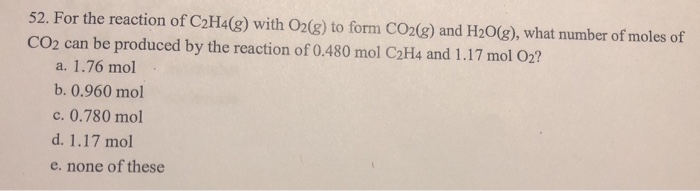52. For the reaction of C2H4(g) with O2(g) to form CO2(g) and H2O(g), what number of moles of CO2 can be produced by the reaction of 0.480 mol C2H4 and 1.17 mol O2? a. 1.76 mol b. 0.960 mol c. 0.780 mol d. 1.17 mol e. none of these

• ### For the reaction of C2H4(g) with O2(g) to form CO2(g) and H2O(g), what number of moles...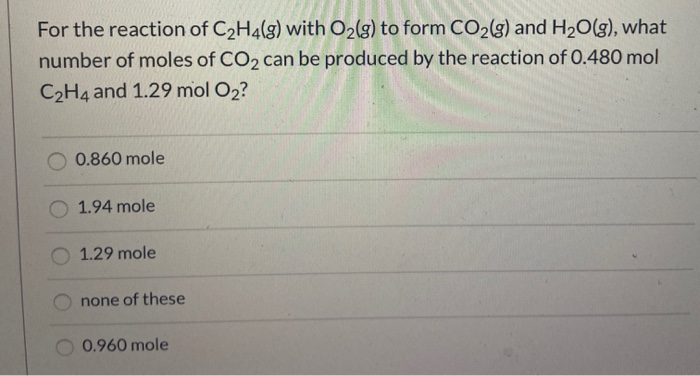For the reaction of C2H4(g) with O2(g) to form CO2(g) and H2O(g), what number of moles of CO2 can be produced by the reaction of 0.480 mol C2H4 and 1.29 mol O2? 0.860 mole 1.94 mole 1.29 mole none of these 0.960 mole

• ### the enthalpy of combustion of CH4(g) to make H2O(l) and CO2(g) is -2340 kJ mol-1. The...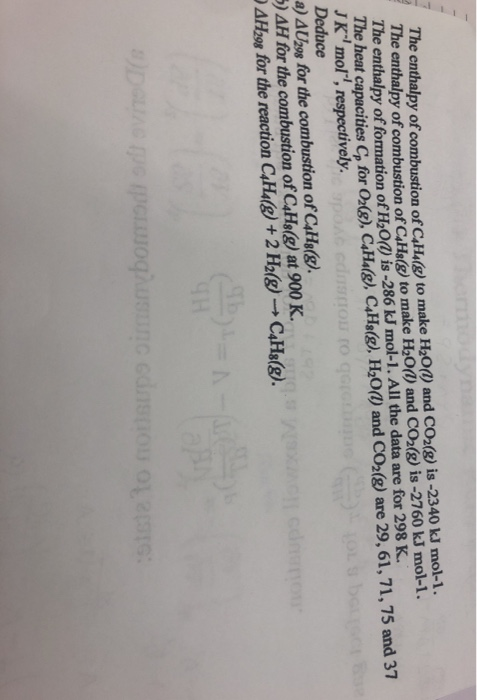the enthalpy of combustion of CH4(g) to make H2O(l) and CO2(g) is -2340 kJ mol-1. The enthalpy of combustion of CH2(g) to make H2O(l) and CO2(g) is -2760 kJ mol-1. The enthalpy of formation of H2O(l) is -286 kJ mol-1. All the data are for 298 K. The heat capacities for O2(g), CHA(8), CH3(g), H2O(l) and CO2(8) are 29, 61, 71, 75 and 37 JK"mor", respectively. Deduce a) 4U298 for the combustion of C4H8(g). 5) AH for the combustion of...

• ### please answer all question and type please Problems and questions 1. Balance the following equations: -...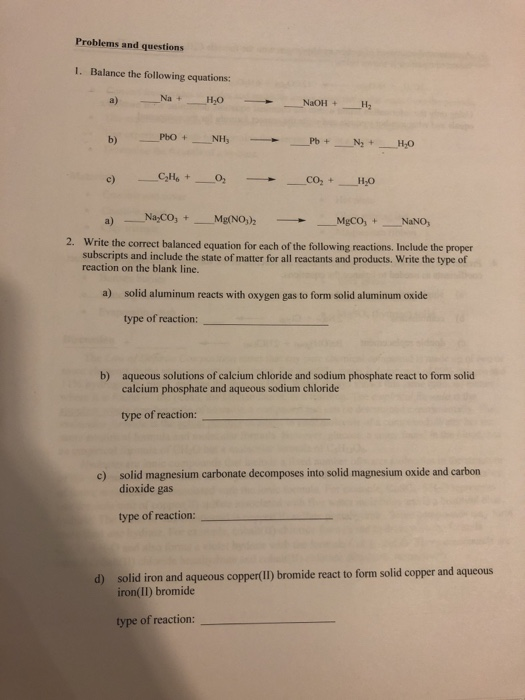please answer all question and type please Problems and questions 1. Balance the following equations: - Na + H₂O NaOH + H2 PbO + _NH Pb + _Ny +_ H_O CH. +_ - _CO+ H2O a) — _NayCO; + _Mg(NO3)2 - > MgCO, + NaNO, 2. Write the correct balanced equation for each of the following reactions. Include the proper subscripts and include the state of matter for all reactants and products. Write the type of reaction on the blank...

• ### 2 C8H18 (l)    +    25 O2 (g) ---->   16 CO2 (g)    +     18 H2O (g) (OR...

2 C8H18 (l)    +    25 O2 (g) ---->   16 CO2 (g)    +     18 H2O (g) (OR 2 C8H18 (l)    +    25 O2 (g) right arrow   16 CO2 (g)    +     18 H2O (g)) Use the following conversions: 1 mol = MW in g (2 d.p.) = 22.414 L All gases are at STP.   How many liters of CO2 would be produced from the reaction of 5.0 L of C8H18 with 55 L of O2? Theoretical yield of CO2 from 5.0 L...